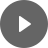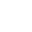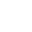5.1 Rates - StudyNinja
Select Page

HSC Standard Maths Resources

Browse: 1. Home  »  5. Rates »  5.1 Rates

5.1 Rates

Basics for this topic are easy to master and it is important you get them right as they will help with complicated questions. There are three things to learn at the basic level:

• Calculate rate
• Conversion between different units

Get access to 20 Mock Exams with over 700 exam-style questions for HSC Standard Maths.

Calculate rate

You are given two measures and need to work out the rate using them. E.g. 10 litres of fuel cost \$14, what is the price per litre?

Example 1

• 10 litres of milk cost \$50. What is the cost per litre of milk?
• Car travels 5,000 km in 80 hours. What is the average speed?
• Total income of 5 employees in company is \$350,000. What is the average income?

This is the second thing you need to know. In this kind of problem, you will be given a rate (or everything you need to calculate one) along with one measure’s overall value and will be required to work back to calculate other measure. E.g. How much distance a car will travel in 5 hours if it travels at 100 km/h? So, rate of 100 km/h is given and overall target value of time of 5 hours is given and need to find distance.

Example 2

Smith earns at the rate of \$15/hour. How long would he need to work to earn \$60?

Conversion between different units

These kinds of problems ask to convert one measure to another. Like convert kilometres to meters, hours to minutes etc. You can expect it be part of a more complicated question where this conversion might be needed to solve other parts of question. Challenge also appears when you have to convert one rate to another rate e.g. km/h to m/s.

Example 3

• Convert 2500 grams to kg
• John travels at 800 m/min. Convert this rate to km/hour

You might find the following videos helpful related to this section:1A Rates (1 of 3)

by Mr Bodgers (click to view channel)

Explains how to convert rates into different units.1A Rates (2 of 3)

by Mr Bodgers (click to view channel)

Explains how to solve rate problems using the unitary method.1A Rates (3 of 3)

by Mr Bodgers (click to view channel)

Explains how to solve rate problems involving speed, distance and time.1B Heart Rate (1 of 3)

by Mr Bodgers (click to view channel)

Introduces heart rate, maximum heart rate (MHR) and target heart rate (THR).1B Heart Rate (2 of 3)

by Mr Bodgers (click to view channel)

Completes an example calculating maximum heart rate (MHR) and target heart rate (THR).1B Heart Rate (3 of 3)

by Mr Bodgers (click to view channel)

Checking a persons health based on their resting heart beat.1C Energy (1 of 4)

by Mr Bodgers (click to view channel)

Explains how to calculate energy costs and defines kilowatt-hours.1C Energy (2 of 4)

by Mr Bodgers (click to view channel)

Completes an example which calculates the energy costs of appliances.1C Energy (3 of 4)

by Mr Bodgers (click to view channel)

Explains what BASIX (building sustainability index) is all about.1C Energy (4 of 4)

by Mr Bodgers (click to view channel)

Explains how to calculate energy costs when you have solar panels.1D Fuel Consumption

by Mr Bodgers (click to view channel)

Explains how to solve problems involving the fuel consumption of cars.There are broadly 5 types of questions you can expect in exam:

Study notes of this section and other resources can be accessed here:

Browse: 1. Home  »  5. Rates »  5.1 Rates